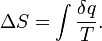# Thermodynamics Entropy change of the universe

Im trying to do an assingment for second year BSc, ive thrown alot of effort into quatum this semester so have fallen a little behind with thermodynamics so help is appreciated!

## Homework Statement

Calculate the change in entrupy of the universe for the following processes
A can of beer, at initial temperature of 4 degrees C, and of heat capacity 2500JK^1 , is allowed to reach thermal equilibrium with its surroundings at 30 degrees C

## Homework Equations

https://www.physicsforums.com/latex_images/24/2495626-1.png [Broken]

Copied the formulas so hoping they have come out alright.

## The Attempt at a Solution

if the can has to increase temperature by 26 degrees K then needs to gain 65000 J of heat energy.

the surroundings must lose 65000J of heat energy so:

65000/277 + 65000/303 = 449.18 J / K

Its not a many mark question but i dont feel confident ive done it right.

Cheers for any help in advance.
Khris

Last edited by a moderator:

Andrew Mason
Homework Helper
You are making two errors.

1. You have to apply the proper sign to the heat flow. The heat flow from the surroundings is negative. The heat flow into the can of beer is positive. You are treating both as positive.

2. The heat flow from the surroundings occurs at constant temperature. BUT the heat flow into the beer does not. The temperature of the beer increases as heat flows into it. So you cannot simply take the heat flow and divide by initial temperature. Work out the expression for temperature of the beer as a function of heat flow in order to calculate the change in entropy.

AM

ok so the loss from the surroundings is correct, but the value for tha can is incorrect i can see that.

i know also the equation:but cant think how to use it.

that equation gives change in entropy as the sum of how the energys changing as the temperature changes is it not? when integrated between the limits of the initial and final temperatures?

Khris

if i use the equation that change in entropy = heat flow / temperature
delta S = Q / T

Where Q = CdT

Then delta S = c Int 1/T between limits T2 and T1 (303 and 277 respectively)

Then i come out with the answer ~ 224 J/K

This any Closer?

Last edited:
Andrew Mason
Homework Helper
that equation gives change in entropy as the sum of how the energys changing as the temperature changes is it not? when integrated between the limits of the initial and final temperatures?

Khris

if i use the equation that change in entropy = heat flow / temperature
delta S = Q / T

Where Q = CdT

Then delta S = c Int 1/T between limits T2 and T1 (303 and 277 respectively)

Then i come out with the answer ~ 224 J/K

This any Closer?
You might show more of your work - like how you get from the integral to the answer. But your answer is correct for the change in entropy of the beer can. So what is the total change in entropy?

AM

im sorry about not including the full calculation, the integral becomes LnT, inbetween the limits it becomes Ln(303/277) multiplied by the specific heat capacity.

So the heat flow into the can of beer is + 224, then the heat flow from the surroundings is negative -214, would i add these together to give the total entropy change of the universe as 438 J/K due to the surroundings losing the 214, and the beer can gaining 224 or would it simply be the differance between these values?

Khris

Andrew Mason
$$\Delta S = \Delta S_{can} + \Delta S_{surr} = 224 -214 = 10 J/K$$